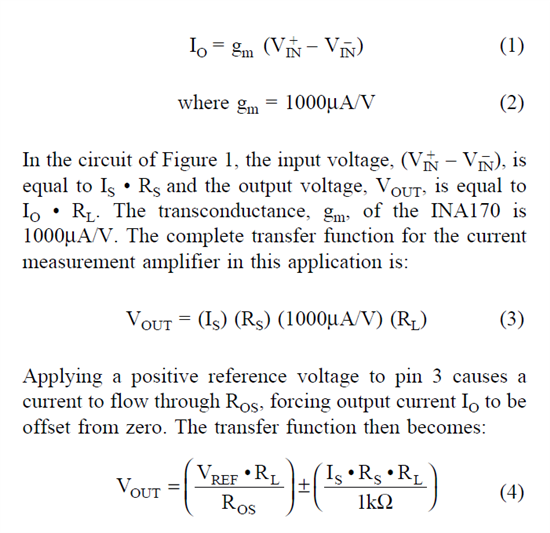If you have a related question, please click the "Ask a related question" button in the top right corner. The newly created question will be automatically linked to this question.

# how to calculate INA170 Io and Vout??

Other Parts Discussed in Thread: INA170

Page 6 of INA170 datasheet, under OPERATION heading there are two equations. I want to know how to derive these two equations and I've tried them myself but cannot find a way to get the answer.

Can I please get some guide?

Thank you.

• Hi Paul,

Not sure which 2 equations you to which you refer, there are actually 4 equations...he is a brief explanation...For equation 1 the Io is the output current (please reference the schematic below for where the output current is flowing).  The gm in equation 1 is the reciprocal of the 1K input resistance inside the INA170.

Equation 2 is just a constant that is provide to you which is nothing more than the reciprocal of the 1K input resistance.

Equation 3 relates the output voltage (which is the output current from equation 1 multiplied by the load resistor RL) to the input voltage.  The input voltage is the current flowing in the shunt resistance multiplied by the shunt resistance, this is the (Is) (Rs) term in the equation.  This (Is) (Rs) term in the equation 3 is equal to the (Vin+ - Vin-) term in equation 1 (assuming ideal conditions).

In equation 4 the second term given, (Is) (Rs) (RL)/(1k) is the exact same term as equation 3...I hope you now see it.  If you perform a circuit analysis from Vref to Vout you will also find that the transfer function is given in the first half of equation 4...that is Vref multiplied by the ratio of RL and Ros will give you the amount of Vout due to Vref...use superposition and the complet output equation 4 is the result.